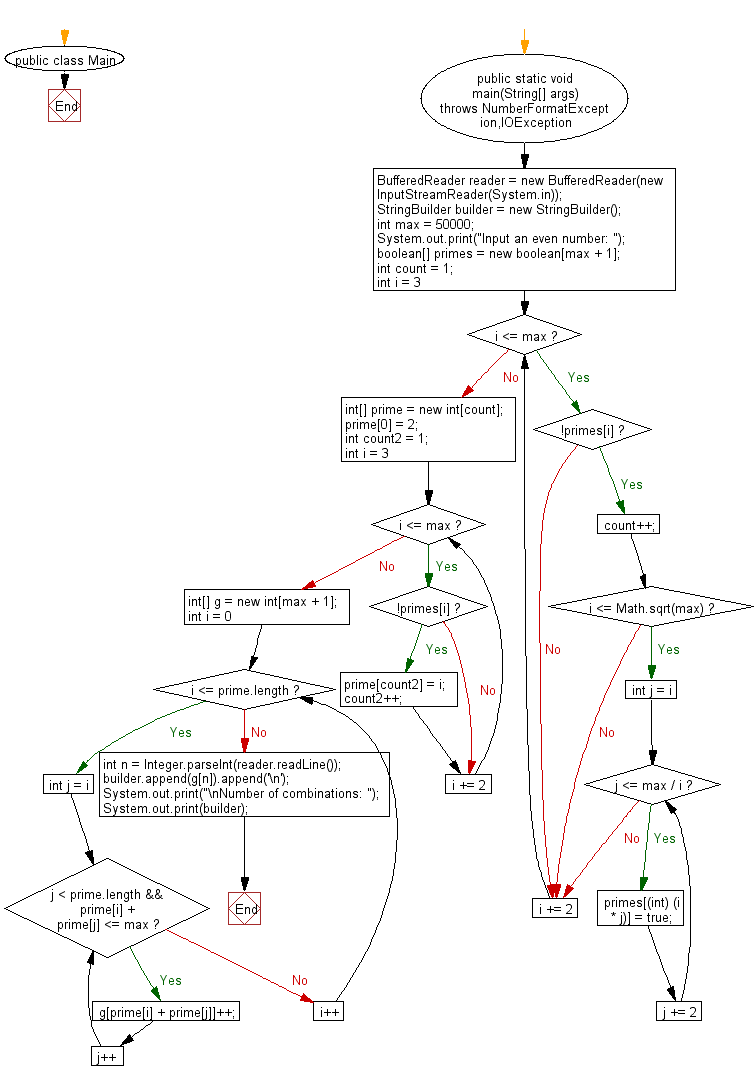# Java Exercises: Accept a even number from the user and create a combinations that express the given number as a sum of two prime numbers

## Java Basic: Exercise-233 with Solution

Write a Java program that accept a even number (n should be greater than or equal to 4 and less than or equal to 50,000, Goldbach number) from the user and create a combinations that express the given number as a sum of two prime numbers. Print the number of combinations.

Goldbach number: A Goldbach number is a positive even integer that can be expressed as the sum of two odd primes. Since four is the only even number greater than two that requires the even prime 2 in order to be written as the sum of two primes, another form of the statement of Goldbach's conjecture is that all even integers greater than 4 are Goldbach numbers.
The expression of a given even number as a sum of two primes is called a Goldbach partition of that number. The following are examples of Goldbach partitions for some even numbers:
6 = 3 + 3
8 = 3 + 5
10 = 3 + 7 = 5 + 5
12 = 7 + 5
...
100 = 3 + 97 = 11 + 89 = 17 + 83 = 29 + 71 = 41 + 59 = 47 + 53

Sample Solution:

Java Code:

`````` import java.io.BufferedReader;
import java.io.IOException;

public class Main {

public static void main(String[] args) throws NumberFormatException,IOException {
StringBuilder builder = new StringBuilder();
int max = 50000;
System.out.print("Input an even number: ");
boolean[] primes = new boolean[max + 1];
int count = 1;
for (int i = 3; i <= max; i += 2) {
if (!primes[i]) {
count++;
if (i <= Math.sqrt(max)) {
for (int j = i; j <= max / i; j += 2) {
primes[(int) (i * j)] = true;
}
}
}
}
int[] prime = new int[count];
prime = 2;
int count2 = 1;
for (int i = 3; i <= max; i += 2) {
if (!primes[i]) {
prime[count2] = i;
count2++;
}
}
int[] g = new int[max + 1];
for (int i = 0; i <= prime.length; i++) {
for (int j = i; j < prime.length && prime[i] + prime[j] <= max; j++) {
g[prime[i] + prime[j]]++;
}
}

builder.append(g[n]).append('\n');
System.out.print("\nNumber of combinations: ");
System.out.print(builder);

}
}
```
```

Sample Output:

```Input an even number: 100

Number of combinations: 6
```

Pictorial Presentation:Flowchart:Java Code Editor:

What is the difficulty level of this exercise?

﻿

## Java: Tips of the Day

Parsing dates:

```import java.io.*;
import java.util.*;
import java.text.*;

String s = "2001/09/23 14:39";

SimpleDateFormat formatter = new SimpleDateFormat ("yyyy/MM/dd H:mm");
Date d = formatter.parse(s, new ParsePosition(0));
```